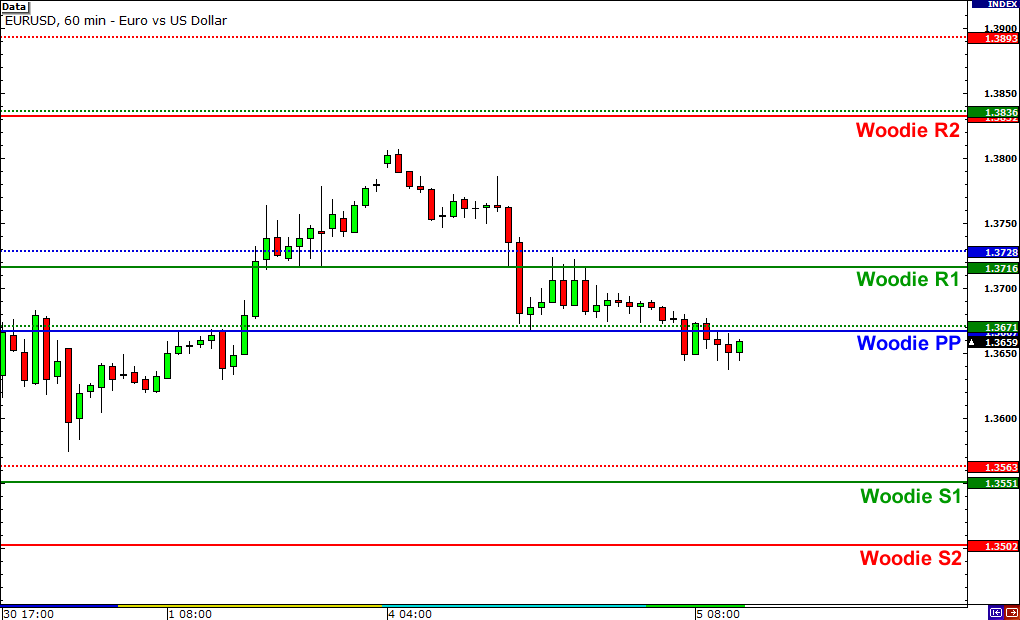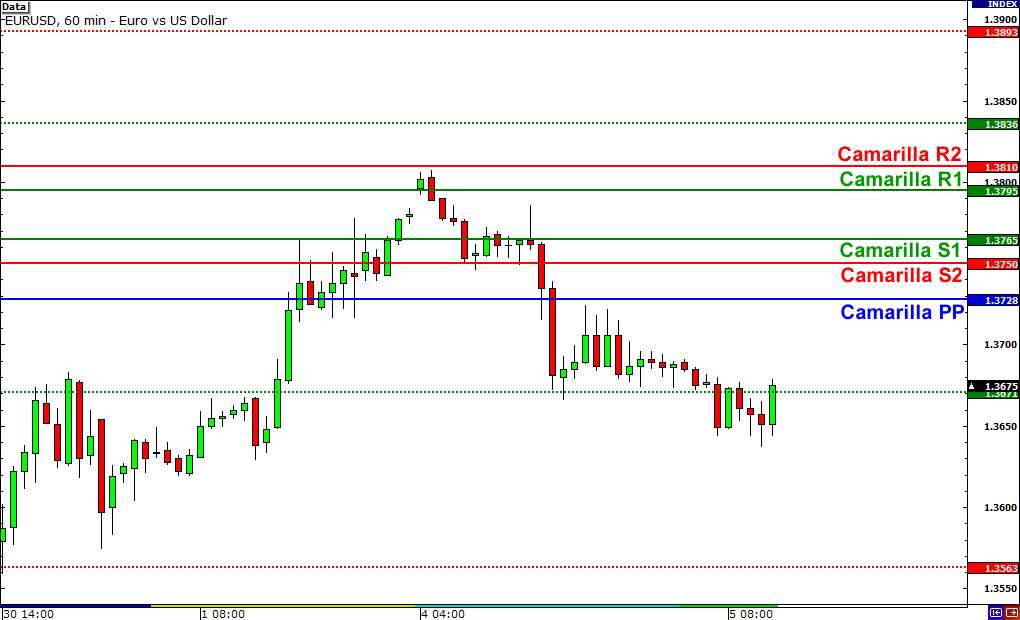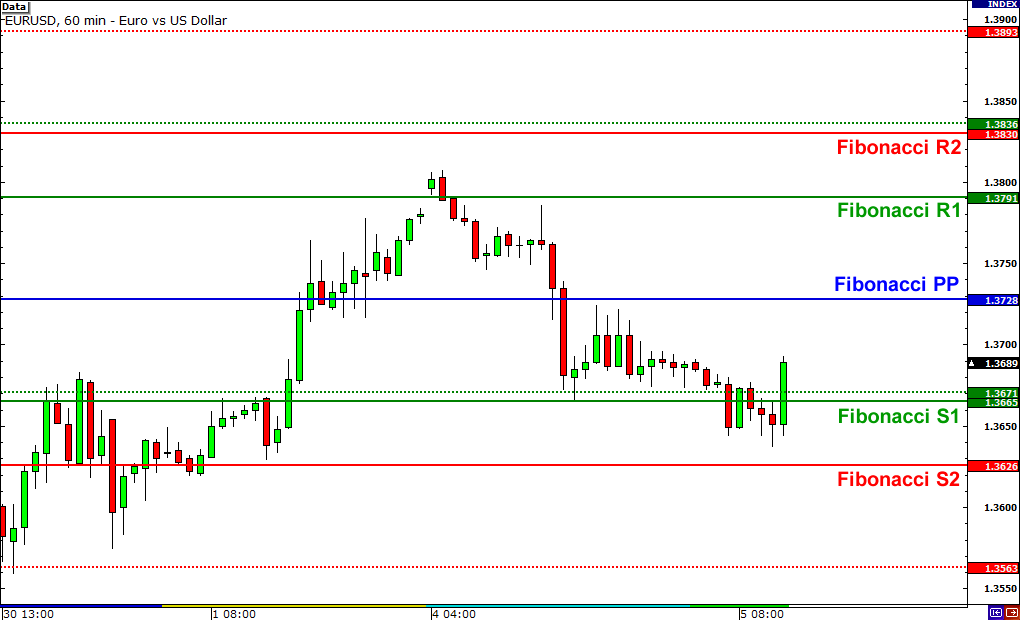While we suggest that you stick to the standard method of calculating pivot points, you should know that there are other ways to calculate for pivot points.

In this lesson, we will talk about these other methods, as well as give you the formulas on how to calculate for these levels.

## Woodie Pivot Point

R2 = PP + High – Low

R1 = (2 X PP) – Low

PP = (H + L + 2C) / 4

S1 = (2 X PP) – High

S2 = PP – High + Low

C – Closing Price, H – High, L – Low

In the formulas above, you’ll notice that the pivot point calculation is very different from the standard method.

Also, in order the calculate for the corresponding support and resistance levels, you would use the difference between the previous day’s high and low, otherwise known as the range.

Here’s a chart example of the Woodie pivot point calculation applied on EURUSD.

The Woodie pivot point, support levels, and resistance levels are the solid lines while the dotted lines represent the levels calculated through the standard method.Because they have different formulas, levels obtained through the Woodie calculations are very different from those gotten through the standard method.

Some traders prefer to use the Woodie formulas because they give more weight to the closing price of the previous period.

Others prefer the standard formulas because many traders make use of those, which could make them self-fulfilling.

In any case, since resistance turns into support (and vice versa), if you choose to use the Woodie formulas, you should keep an eye on these levels as they could become areas of interest. Whatever floats your boat!

## Camarilla Pivot Point

R4 = C + ((H-L) x 1.5000)

R3 = C + ((H-L) x 1.2500)

R2 = C + ((H-L) x 1.1666)

R1 = C + ((H-L) x 1.0833)

PP = (H + L + C) / 3

S1 = C – ((H-L) x 1.0833)

S2 = C – ((H-L) x 1.1666)

S3 = C – ((H-L) x 1.2500)

S4 = C – ((H-L) x 1.5000)

C – Closing Price, H – High, L – Low

The Camarilla formulas are similar to the Woodie formula. They also use the previous day’s close and range to calculate the support and resistance levels.

The only difference is that you should calculate for 8 major levels (4 resistance and 4 support), and each of these levels should be multiplied by a multiplier.

The main concept of Camarilla pivot points is that it is based on the idea that price has a natural tendency to revert back to the mean (sound familiar?), or in this case, the previous day’s close.

The idea is that you should buy or sell when price reaches either the third support or resistance level.

However, if price were to burst through S4 or R4, it would mean that the intraday trend is strong, and it’s about time you jump on that bandwagon!

Check out how the Camarilla calculation gives different levels (solid lines) compared to the standard method’s levels (dotted lines)!As you can see from the chart above, more emphasis is given to the closing price as opposed to the pivot point.

Because of this, it’s possible that resistance levels could be below the pivot point or support levels could be above it.

See how all the support and resistance levels are above the Camarilla pivot point?

## Fibonacci Pivot Point

R3 = PP + ((High – Low) x 1.000)

R2 = PP + ((High – Low) x .618)

R1 = PP + ((High – Low) x .382)

PP = (H + L + C) / 3

S1 = PP – ((High – Low) x .382)

S2 = PP – ((High – Low) x .618)

S3 = PP – ((High – Low) x 1.000)

C – Closing Price, H – High, L – Low

Fibonacci pivot point levels are determined by first calculating the pivot point like you would the standard method.

Next, multiply the previous day’s range with its corresponding Fibonacci level. Most traders use the 38.2%, 61.8% and 100% retracements in their calculations.

Finally, add or subtract the figures you get to the pivot point and voila, you’ve got your Fibonacci pivot point levels!

Look at the chart below to see how the levels calculated through the Fibonacci method (solid lines) differ from those calculated through the standard method (dotted lines).The logic behind this is that many traders like using the Fibonacci ratios. People use it for retracement levels, moving averages, etc.

Why not use it for pivot points as well?

Remember that both Fibonacci and pivot points levels are used to find support and resistance. With so many traders looking at these levels, they can actually become self-fulfilling.

## Which pivot point method is best?

The truth is, just like all the variations of all the other indicators that you’ve learned so far, there is no single best method.

It really all depends on how you combine your knowledge of pivot points with all the other tools in your forex trading toolbox.

Just know that most charting software that do automatic calculations normally use the standard method in calculating for the pivot point levels.

But now that you know how to calculate for these levels on your own, you can give them all a swing and see which one works best for you. Pivot away!